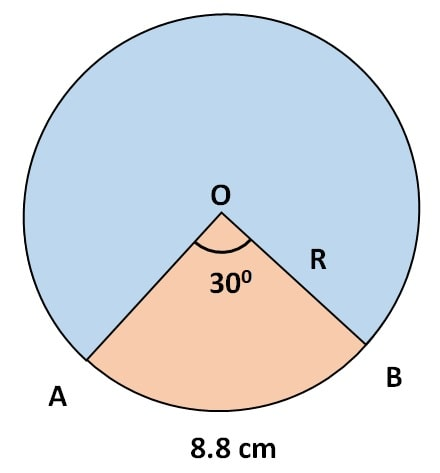Q) A pendulum swings through an angle of 30and describes an arc of 8.8 cm in length. Find the length of the pendulum.

Ans: Let’s draw a diagram and plot the given information.

(Note: your question will become clearer and your answer will never be wrong if you draw a diagram)We know that the circumference of a circle is = 2 π R

And length of an arc making angle of θ at centre = 2 π RHere, we are given value of θ = 300 and arc length = 8.8 cm,

∴ 8.8 = 2 xx R x8.8 = 2 xx r x= R xR == 16.8

Therefore the arc length is 16.8 cm.

Scroll to Top